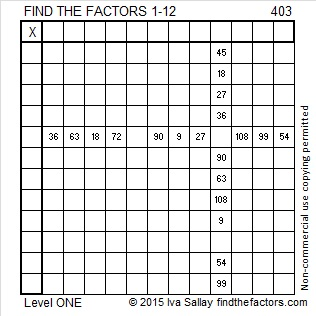# 403 and Level 1

Madam, I’m Adam! There is something palindromic about the factors of 403. What could they possibly be? Scroll down past the puzzle to see what they are.Print the puzzles or type the factors on this excel file: 12 Factors 2015-02-23

• 403 is a composite number.
• Prime factorization: 403 = 13 x 31
• The exponents in the prime factorization are 1 and 1. Adding one to each and multiplying we get (1 + 1)(1 + 1) = 2 x 2 = 4. Therefore 403 has exactly 4 factors.
• Factors of 403: 1, 13, 31, 403
• Factor pairs: 403 = 1 x 403 or 13 x 31
• 403 has no square factors that allow its square root to be simplified. √403 ≈ 20.0749